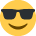# Practice assessment 1 - Question 1 (b)

Hi,

I am having complete mind block on how to work out the total fixed and variable overheads for the below question! Please can someone help and explain?

Question is below:
Units produced: 19,000 - £295,000
Units produced: 23,900 - £324,400

So asks you to calculate the below based on above:
Fixed £ Variable £
21,000
36,000

Please can someone help me on the above?

Thank you## Comments

• Firstly, we can work out the variable unit cost by using the high-low method. Doing this, we get 4,900 units at £29,400. Divide £29,400 by 4,900 to get the variable unit cost, which would be £6.00.

We can now use this to find the fixed cost. We'll use the 19,000 example. £6.00 * 19,000 is £114,000. Subtract this from £295,000 to find the fixed cost, £181,000. You can replicate this in the 23,900 example too.

So for the calculations for 21,000 and 36,000, the fixed cost would be £181,000, since it doesn't vary based on units produced. Variable costs you just multiply the variable unit cost (£6.00) by the units produced, which equates to £126,000 and £216,000 respectively.

Total costs would, therefore, be £307,000 and £397,000 respectively.

Let me know if something doesn't make sense. Welcome to the AAT forumsMatthew Dearlove MAAT
Currently on CIMA Management Level. Passed P2 and F2, studying E2.
• Hello,

Ok so first of all we will have to apply the High-Low costing method, this is because we need to find out variable and fixed costs, we have 2 period figures so we can easily work it out by doing High-Low method;

You need to take the highest units for 1 period – lowest units for 1 period, in this instance is £23,900 - £19,000 = £4,900
Then you look at the total costs, again same principle highest cost – lowest cost; £324,400 - £295,000 = £29,400
Now you simply divide total costs by units; £29,400 / £4,900 = £6.00 – This is variable cost
To check if we are correct;
£6.00 x 23,900 = £143,400. £324,400 - £143,400 = £181,000 Fixed costs
£6.00 x 19,000 = £114,000. £295,000 - £143,400 = £181,000 Fixed Costs
From this we can now see that £6.00 is our variable cost per unit and £181,000 is our fixed costs;

21,000 Units;
£6.00 x 21,000 = £126,000 Variable Cost + £181,000 Fixed Cost = £307,000 Total Cost

36,000 Units;
£6.00 x £36,000 = £216,000 Variable Cost + £181,000 Fixed Cost = £397,000 Total Cost

I hope this helps!
Kind Regards,

Norvydas Valavicius.
Sign In or Register to comment.